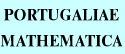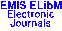PORTUGALIAEMATHEMATICA Vol. 56, No. 3, pp. 329-343 (1999)

Previous Article

Next Article

Contents of this Issue

Other Issues

ELibM Journals

ELibM Home

EMIS Home

## Rational Arithmetical Functions of Order (2,1) with Respect to Regular Convolutions

### Pentti Haukkanen

Department of Mathematical Sciences, University of Tampere,
P.O. Box 607, FIN-33101 Tampere - FINLAND

Abstract: S.S. Pillai's arithmetical function $P(n)=\sum_{m\ppmod n}(m,n)$ is an example of a rational arithmetical function of order $(2,1)$. We generalize $P(n)$ with respect to Narkiewicz's regular convolution and show that the generalized Pillai's function is an example of a rational arithmetical function of order $(2,1)$ with respect to Narkiewicz's regular convolution. We derive identities for rational arithmetical functions of order $(2,1)$ with respect to Narkiewicz's regular convolution and therefore also for Pillai's function and its generalization.

Keywords: Rational arithmetical functions; Narkiewicz's regular convolution; Pillai's function; identical equations.

Classification (MSC2000): 11A25.

Full text of the article: